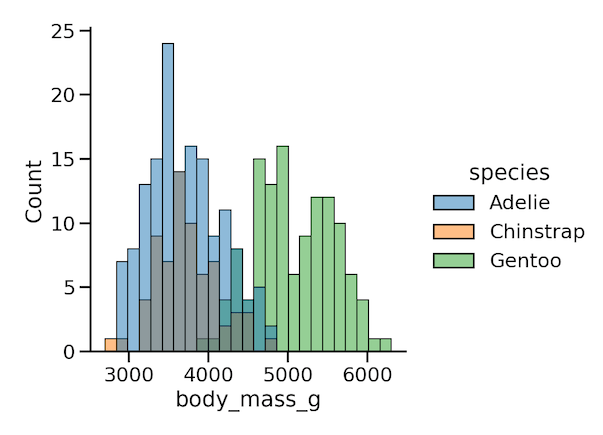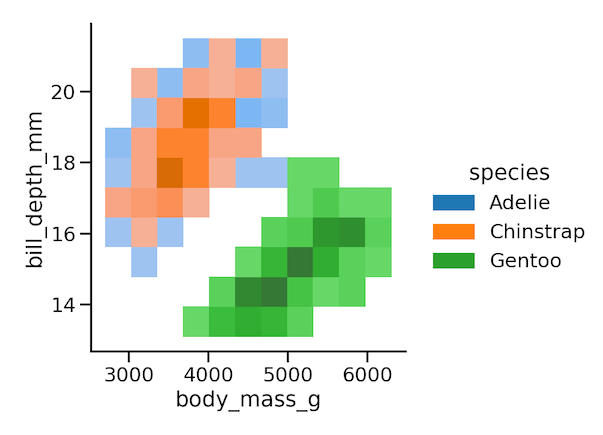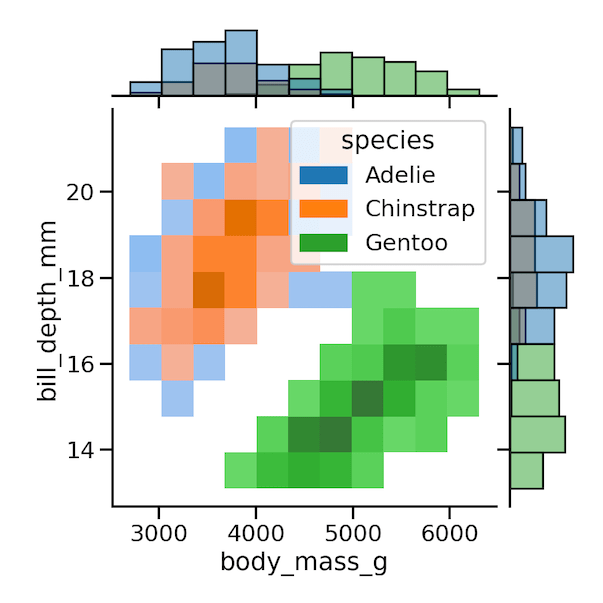## Tuesday, September 15, 2020

### Seaborn Version 0.11.0 is here with displot, histplot and ecdfplotSeaborn Version 0.11 is Here

Seaborn, one of the data visualization libraries in Python has a new version, Seaborn version 0.11, with a lot of new updates. One of the biggest changes is that Seaborn now has a beautiful logo. Jokes apart, the new version has a lot of new things to make data visualization better. This is a quick blog post covering a few of the Seaborn updates.

### displot() for univariate and bivariate distributions

One of the big new changes is “Modernization of distribution functions” in Seaborn version 0.11. The new version of Seaborn has three new functions displot(), histplot() and ecdfplot() to make visualizing distributions easier. Yes, we don’t have to write your own function to make ECDF plot any more.

Seaborn’s displot() can be used for visualizing both univariate and bivariate distributions. Among these three new function, displot function gives a figure level interface to the common distribution plots in seaborn including histograms (histplot), density plots, empirical distributions (ecdfplot), and rug plots. For example, we can use displot() and create

• histplot() with kind=”hist” (this is default)
• kdeplot() (with kind=”kde”)
• ecdfplot() (with kind=”ecdf”)
• We can also add rugplot() to show the actual values of the data to any of these plots.

Don’t get confused with distplot() for displot(). displot() is the new distplot() with better capabilities and distplot() is deprecated starting from this Seaborn version.

With the new displot() function in Seaborn, the plotting function hierarchy kind of of looks like this now covering most of the plotting capabilities.Searborn Plotting Functions Hierarchy

In addition to catplot() for categorical variables and relplot() for relational plots, we now have displot() covering distributional plots.

Let us get started trying out some of the functionalities. We can install the latest version of Seaborn

```pip install seaborn
```

Let us load seaborn and make sure we have Seaborn version 0.11.

```import seaborn as sns
print(sns.__version__)
0.11.0
```

We will use palmer penguin data set to illustrate some of the new functions and features of seaborn. Penguins data is readily available as part of seaborn and we can load using load_dataset() function.

```penguins = sns.load_dataset("penguins")
```
```penguins.head()
species island  bill_length_mm  bill_depth_mm   flipper_length_mm       body_mass_g     sex
0       Adelie  Torgersen       39.1    18.7    181.0   3750.0  Male
1       Adelie  Torgersen       39.5    17.4    186.0   3800.0  Female
2       Adelie  Torgersen       40.3    18.0    195.0   3250.0  Female
3       Adelie  Torgersen       NaN     NaN     NaN     NaN     NaN
4       Adelie  Torgersen       36.7    19.3    193.0   3450.0  Female
```

We can create histograms with Seaborn’s histplot() function, KDE plot with kdeplot() function, and ECDF plot with ecdfplot(). However, we primarily use displot() to illustrate Seaborn’s new capabilities.

### Histograms with Seaborn displot()

Let us make a simple histogram with Seaborn’s displot() function.

```plt.figure(figsize=(10,8))
sns.displot(penguins,
x="body_mass_g",
bins=25)
plt.savefig("Seaborn_histogram_with_displot.png",
format='png',dpi=150)
```

Here we have also specified the number of bins in the histogram.Seaborn histogram with displot()

We can also color the histogram by a variable and create overlapping histograms.
```plt.figure(figsize=(10,8))
sns.displot(penguins,
x="body_mass_g",
hue="species",
bins=25)
plt.savefig("Seaborn_overlapping_histogram_hue_with_displot.png",
format='png',dpi=150)
```

In this example, we color penguins’ body mass by species.Seaborn displot(): overlapping histograms using hue

### Facetting with Seaborn displot()

With “col” argument we can create “small multiples” or faceting to create multiple plots of the same type using subsets of data based on a variable’s value.

```plt.figure(figsize=(10,8))
sns.displot(penguins,
x="body_mass_g",
col="species",
bins=25)
plt.savefig("Seaborn_facetting_histogram_col_with_displot.png",
format='png',dpi=150)
```

Here, we have facetted by values of penguins’ species in our data set.Seaborn displot(): facetting histogram using col

### Density plot with Seaborn’s displot()

Let us use displot() and create density plot using kind=”kde” argument. Here we also color by species variable using “hue” argument.

```plt.figure(figsize=(10,8))
sns.displot(penguins,
x="body_mass_g",
hue="species",
kind="kde")
plt.savefig("Seaborn_kernel_density_plot_with_displot.png",
format='png',dpi=150)
```Seaborn displot(): kernel density plots

Check out the Seaborn documentation, the new version has a new ways to make density plots now.

### ECDF Plot with Seaborn’s displot()

One of the personal highlights of Seaborn update is the availability of a function to make ECDF plot. ECDF aka Empirical Cumulative Distribution is a great alternate to visualize distributions.

In an ECDF plot, x-axis correspond to the range of data values for variables and on the y-axis we plot the proportion of data points (or counts) that are less than are equal to corresponding x-axis value.

Unlike histograms and density plot, ECDF plot enables to visualize the data directly without any smoothing parameters like number of bins. Its use possibly visible when you have multiple distributions to visualize.

the relationship between the appearance of the plot and the basic properties of the distribution (such as its central tendency, variance, and the presence of any bimodality) may not be as intuitive.

Let us make ecdf plot using displot() using kind=”ecdf”. Here we make ecdf plot of a variable and color it based on values of another variable.

```plt.figure(figsize=(10,8))
sns.displot(penguins,
x="body_mass_g",
hue="species",
kind="ecdf")
plt.savefig("Seaborn_ecdf_plot_with_displot.png",
format='png',dpi=150)
```Seaborn displot(): Empirical Cumulative Density Function (ECDF) Plot

### Bivariate KDE plot and Histogram with displot()

With kdeplot(), we can also make bivariate density plot. In this example, we use displot() with “kind=’kde'” to make bivariate density/ contour plot.

```plt.figure(figsize=(10,8))
sns.displot(data=penguins,
x="body_mass_g",
y="bill_depth_mm",
kind="kde",
hue="species")
plt.savefig("Seaborn_displot_bivariate_kde_contour.png",
format='png',dpi=150)
```Seaborn displot(): bivariate KDE Density plot

We can also make bivariate histogram with displot() using kind=”hist” option or histplot() to make density plot.

```plt.figure(figsize=(10,8))
sns.displot(data=penguins,
x="body_mass_g",
y="bill_depth_mm",
kind="hist",
hue="species")
plt.savefig("Seaborn_displot_bivariate_hist.png",
format='png',dpi=150)
```Seaborn displot() Bivariate histogram

### New features to Seaborn jointplot()

With Seaborn 0.11, jointplot also has gained some nice features. Now jointplot() can take “hue” as argument to color data points by a variable.

```sns.jointplot(data=penguins,
x="body_mass_g",
y="bill_depth_mm",
hue="species")
```Seaborn jointplot color by variable using “hue”

And jointplot() also gets a way to plot bivariate histogram on the joint axes and univariate histograms on the marginal axes using kind=”hist” argument to jointplot().

```sns.jointplot(data=penguins,
x="body_mass_g",
y="bill_depth_mm",
hue="species",
kind="hist")
```Seaborn jointplot color by variable: bivariate histogram

Another big change that will help writing better code to make data visualization is that most Seaborn plotting functions, will now require their parameters to be specified using keyword arguments. Otherwise, you will see FutureWarning in v0.11.

As part of the update, Seaborn has also got spruced up documentation for Seaborn’s capabilities. Check out the new documentation on data structure that is accepted by Seaborn plotting functions. Some of the functions can take the data in both wide and long forms of data. Currently, the distribution and relational plotting functions can handle both and in future releases other Seaborn functions also will get the same data inputs.

The post Seaborn Version 0.11.0 is here with displot, histplot and ecdfplot appeared first on Python and R Tips.

from Python and R Tips https://ift.tt/2RscPUT
via Gabe's MusingsGabe's Musings